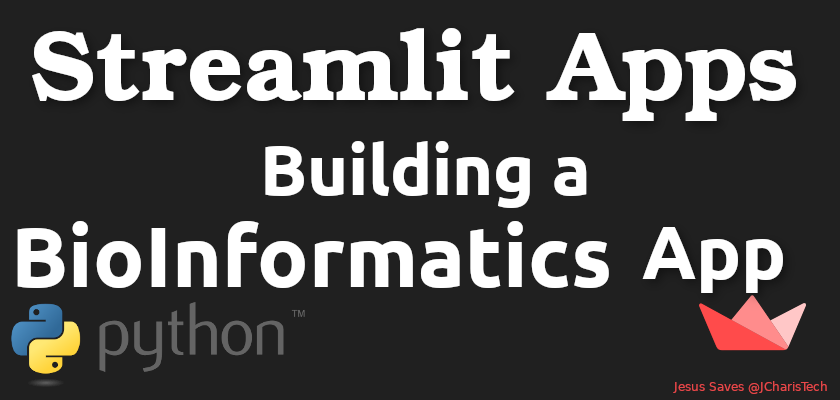# Building A Simple Bioinformatics App with Streamlit and BioPython

Bioinformatics which is also called Biological Data Science is an awesome and fascinating field of  science.

In this tutorial we will build a simple app using Streamlit to do some basic sequence analysis and dot plot of two DNA sequences.

We will be using BioPython and NeatBio to do our processing of our sequence and then utilize Streamlit for our UI.

Let us first install our necessary packages

`pip install biopython neatbio streamlit`

The Structure of our App

Our simple app will have two main sections

• DNA Sequence: where we analyse our sequence from a FASTA file
• DotPlot: where we compare two different sequences and generate a dotplot

In designing the first section for the DNA Sequence we will use the file_uploader or drag and drop feature of streamlit and then process our FASTA file using BioPython and NeatBio. We will be restricting the files to only FASTA files that is files with .fasta or .fa extensions.

To get a more elaborate tutorial on BioPython you can check out this course.

We will then do the basics such as

• DNA Composition (GC content,AT content)
• Transcription
• Translation
• Plot our nucleotide frequency
• Amino acid frequency

In the second part will compare two sequences and generate a dotplot for them. In generating a dotplot will be using a custom function as well as matplotlib to produce a beautiful dotplot.

Below is the entire code for the app.

``````import streamlit as st
import matplotlib.pyplot as plt
import matplotlib
matplotlib.use("Agg")
from Bio.Seq import Seq
from Bio import SeqIO
from collections import Counter
import neatbio.sequtils as utils
import numpy as np
from PIL import Image

def delta(x,y):
return 0 if x == y else 1

def M(seq1,seq2,i,j,k):
return sum(delta(x,y) for x,y in zip(seq1[i:i+k],seq2[j:j+k]))

def makeMatrix(seq1,seq2,k):
n = len(seq1)
m = len(seq2)
return [[M(seq1,seq2,i,j,k) for j in range(m-k+1)] for i in range(n-k+1)]

def plotMatrix(M,t, seq1, seq2, nonblank = chr(0x25A0), blank = ' '):
print(' |' + seq2)
print('-'*(2 + len(seq2)))
for label,row in zip(seq1,M):
line = ''.join(nonblank if s < t else blank for s in row)
print(label + '|' + line)

def dotplot(seq1,seq2,k = 1,t = 1):
M = makeMatrix(seq1,seq2,k)
plotMatrix(M, t, seq1,seq2) #experiment with character choice

# Convert to Fxn
def dotplotx(seq1,seq2):
plt.imshow(np.array(makeMatrix(seq1,seq2,1)))
# on x-axis list all sequences of seq 2
xt=plt.xticks(np.arange(len(list(seq2))),list(seq2))
# on y-axis list all sequences of seq 1
yt=plt.yticks(np.arange(len(list(seq1))),list(seq1))
plt.show()

def gc_content(seq):
result = float(str(seq).count('G') + str(seq).count('C'))/len(seq) * 100
return result

def at_content(seq):
result = float(str(seq).count('A') + str(seq).count('T'))/len(seq) * 100
return result

def main():
"""A Simple Streamlit App """
st.title("BioInformatics App")

choice = st.sidebar.selectbox("Select Activity",activity)
if choice == 'Intro':
elif choice == "DNA Sequence":

if seq_file is not None:
# st.write(dna_record)
dna_seq = dna_record.seq

if details == "Description":
st.write(dna_record.description)
elif details == "Sequence":
st.write(dna_record.seq)

# Nucleotide Frequencies
dna_freq = Counter(dna_seq)
st.write(dna_freq)
thymine_color = st.beta_color_picker("thymine Color")
guanine_color = st.beta_color_picker("Guanine Color")
cytosil_color = st.beta_color_picker("cytosil Color")

if st.button("Plot Freq"):
barlist = plt.bar(dna_freq.keys(),dna_freq.values())
barlist.set_color(thymine_color)
barlist.set_color(guanine_color)
barlist.set_color(cytosil_color)

st.pyplot()

gc_score = utils.gc_content(str(dna_seq))
at_score = utils.at_content(str(dna_seq))
st.json({"GC Content":gc_score,"AT Content":at_score})

# Nucleotide Count
nt_count = st.text_input("Enter Nucleotide Here","Type Nucleotide Alphabet")
st.write("Number of {} Nucleotide is ::{}".format((nt_count),str(dna_seq).count(nt_count)))

# Protein Synthesis
p1 = dna_seq.translate()
aa_freq = Counter(str(p1))

if st.checkbox("Transcription"):
st.write(dna_seq.transcribe())

elif st.checkbox("Translation"):
st.write(dna_seq.translate())

elif st.checkbox("Complement"):
st.write(dna_seq.complement())

elif st.checkbox("AA Frequency"):
st.write(aa_freq)

elif st.checkbox("Plot AA Frequency"):
aa_color = st.beta_color_picker("Pick An Amino Acid Color")
# barlist = plt.bar(aa_freq.keys(),aa_freq.values(),color=aa_color)
# barlist.set_color(aa_color)
plt.bar(aa_freq.keys(),aa_freq.values(),color=aa_color)
st.pyplot()

elif st.checkbox("Full Amino Acid Name"):
aa_name = str(p1).replace("*","")
aa3 = utils.convert_1to3(aa_name)
st.write(aa_name)
st.write("=====================")
st.write(aa3)

st.write("=====================")
st.write(utils.get_acid_name(aa3))

# Top Most Common Amino

elif choice == "DotPlot":
st.subheader("Generate Dot Plot For Two Sequences")

if seq_file1 and seq_file2 is not None:
# st.write(dna_record)
dna_seq1 = dna_record1.seq
dna_seq2 = dna_record2.seq

if details == "Description":
st.write(dna_record1.description)
st.write("=====================")
st.write(dna_record2.description)
elif details == "Sequence":
st.write(dna_record1.seq)
st.write("=====================")
st.write(dna_record2.seq)

cus_limit = st.number_input("Select Max number of Nucleotide",10,200,50)
if st.button("Dot Plot"):
st.write("Comparing the first {} Nucleotide of the Two Sequences".format(cus_limit))
dotplotx(dna_seq1[0:cus_limit],dna_seq2[0:cus_limit])

st.pyplot()

if __name__ == '__main__':
main()

``````

You can also check out the entire video tutorial below

Thanks for watching

Jesus Saves

By Jesse E. Agbe(JCharis)

### 2 thoughts on “Building A Simple Bioinformatics App with Streamlit and BioPython”

1.I love your blog.. very nice colors & theme. Did you create this website yourself or did you hire someone to do it for you? Plz respond as I’m looking to create my own blog and would like to know where u got this from. thank you

1.jesse_jcharis

Thanks for the kind words. We did it ourselves.